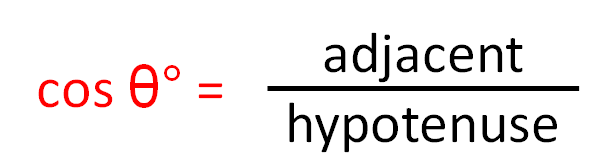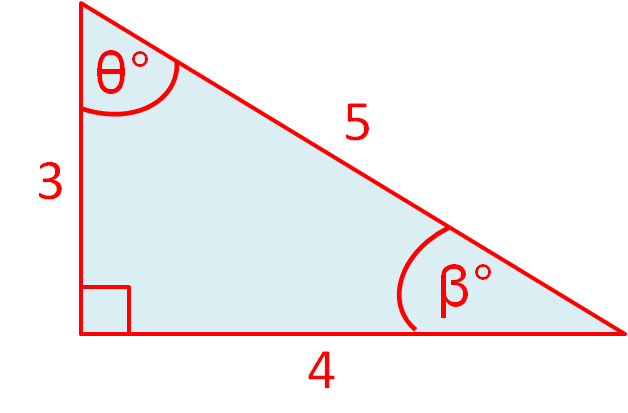• ## Accessibility

Open the accessibility toolbar to change fonts and contrast, access a dictionary, use a ruler and more

In a right-angled triangle, the sides and the angles are connected by three trigonometric ratios.The three trig ratios are:A quick way to remember this is to memorise the word

SOHCAHTOA

Example

Use the side lengths of this triangle to state the value of cosθº ?Label the sides adjacent, opposite and hypotenuse in relation to the angle θº.SOHCAHTOA

The side adjacent to the angle θ has length 3.

The hypotenuse has length 5.

The correct trig ratio is COS so cos θº= 3/5

10 questions